# Community

Blog Events Webinars Tutorials Forum
×
Community Blog How Do Frontend Developers Learn Functional Programming?

# How Do Frontend Developers Learn Functional Programming?

This article explains ways to learn functional programming through a list of tips and tricks.By Xulun from F(x) Team

Functional programming has been around for nearly 60 years. Since the birth of the Lisp language in the 1960s, its different dialects have emerged one after another. While its dialects have brought a thriving ecology, there is also trouble with compatibility. Therefore, various standardization efforts have been constantly done to organize according to the current implementation. For example, Lisp defines the Common Lisp specification, but a large branch of scheme is an independent one. Another functional language ML was standardized to Standard ML later, but it could not stop another dialect, ocaml. Then, a committee was founded in practice to define a general functional programming language, which is Haskell. Later, Haskell was considered by functional fundamentalists to be a purely functional language, while Lisp and ML did not completely conform to the purely functional language principles.

Regardless of purity, performance issues have always affected the widespread use of functional programming languages. Until the single-core performance reached its peak in the Pentium 4 era, and mproving single-thread performance was gone, functional programming languages became popular again because of their multithreading security. Erlang emerged first, followed by Scala and Clojure.

The idea of functional programming has also been affecting traditional programming languages. For instance, Java 8 began to support Lambda expressions, and the skyscrapers of functional programming were originally built on Lambda computing.

However, backend Java developers consider the idea of functional programming optional, while frontend developers say it is a necessary option.

## 1. Why Do Frontend Developers Need to Learn the Idea of Functional Programming?

The components of the React framework supported class-based components, and functional components very early. For example, the following method of class inheritance is more understandable for most developers that have learned the object-oriented programming idea:

``````class Welcome extends React.Component {
render() {
return <h1>Hello, {this.props.name}</h1>;
}
}``````

However, it can be written as a functional component:

``````function Welcome(props) {
return <h1>Hello, {props.name}</h1>;
}``````Starting from React 16.8, the emergence of React Hooks has made functional programming ideas more indispensable.

For example, we can add a state to the functional component through React Hooks:

``````import React, { useState } from 'react';

function Example() {
// Declare a new state variable, which we'll call "count"
const [count, setCount] = useState(0);

return (
<div>
<p>You clicked {count} times</p>
<button onClick={() => setCount(count + 1)}>
Click me
</button>
</div>
);
}``````

Similarly, we can use useEffect to handle lifecycle-related operations, which is equivalent to processing ComponentDidMount:

``````import React, { useState, useEffect } from 'react';

function Example() {
const [count, setCount] = useState(0);

// Similar to componentDidMount and componentDidUpdate:
useEffect(() => {
// Update the document title using the browser API
document.title = `You clicked \${count} times`;
});

return (
<div>
<p>You clicked {count} times</p>
<button onClick={() => setCount(count + 1)}>
Click me
</button>
</div>
);
}``````

What is the relationship between APIs, such as useState, useEffect, and functional programming?

We can look at the API documentation of useEffect:

Mutations, subscriptions, timers, logging, and other side effects are not allowed inside the main body of a function component (referred to as React's render phase). Doing so will lead to confusing bugs and inconsistencies in the UI.

Instead, use useEffect.The function passed to useEffect will run after the render is committed to the screen. Think of effects as an escape hatch from React's purely functional world into the imperative world.

Mutations, subscriptions, timers, logging, and other side effects are not allowed to be used during the rendering of functional components. useEffect is the channel between React's purely functional world and the imperative world.

When we have finished writing the frontend with React, we want to write a BFF functionality. We find that Serverless has also changed from the original framework with nesting classes (as a nesting doll) to the one that only needs one function for a functionality. The following is an official example of the Alibaba Cloud Serverless HTTP function:

``````var getRawBody = require('raw-body')
module.exports.handler = function (request, response, context) {
for (var key in reqHeader) {
};

// get request info
var url = request.url
var path = request.path
var queries = request.queries
var queryStr = ''
for (var param in queries) {
queryStr += param + "=" + queries[param] + '  '
};
var method = request.method
var clientIP = request.clientIP

// get request body
getRawBody(request, function (err, data) {
var body = data
// you can deal with your own logic here

// set response
var respBody = new Buffer('requestHeader:' + headerStr + '\n' + 'url: ' + url + '\n' + 'path: ' + path + '\n' + 'queries: ' + queryStr + '\n' + 'method: ' + method + '\n' + 'clientIP: ' + clientIP + '\n' + 'body: ' + body + '\n')
response.setStatusCode(200)
response.send(respBody)
})
};``````

There is no need to pay attention to side effects and other requirements since it is written with functions. It is better to use functional ideas rather than imperative ones.

## 2. The Methods and Misunderstandings of Learning Functional Programming

If you search how to learn functional programming on the Internet, nine times out of ten, people will recommend starting with Haskell to learn functional programming.

Then, you probably will learn the famous saying, "A monad is just a monoid in the category of endofunctors, what's the problem?"

The translation seems to be useless, "A monad is just a monoid in the category of self-function."

Don't be frightened by these terms. React provides Hooks, such as useState and useEffect, which are tools to help us solve side-effect operations outside the purely functional world. Similarly, the Functor and Monad are also tools or can be considered as design patterns.

The importance of Monad in Haskell is that for operations, such as I/O, which are basic but have side effects, Haskell of pure functions cannot be processed by functional methods, so I/O Monad is needed. Most of the other languages are not so pure, so their side effect operations, such as I/O, can be solved through non-functional methods. Therefore, the sentence above is considered the secret code of the Haskell user group.

We will understand functional programming from a higher level with background knowledge, such as category theory and type theory. However, it may be better for most frontend developers to learn how to use functional programming before picking up the background knowledge. The frontend development has a relatively short plan, and it is difficult to find a long period to learn. However, we can make iterative progress, and we will reach the same goal eventually.

It is better to practice the pattern well and use it in the code to solve practical business problems than stay in the imperative world, frightened by difficulties.

## 3. The Essence of Functional Programming: Free of Side Effects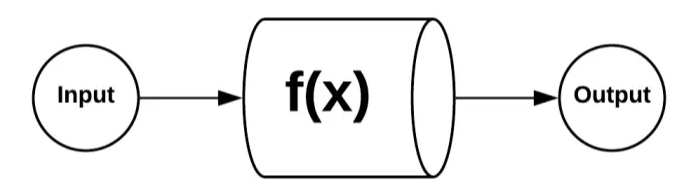React Hooks has already put the side effects in front of us, which is an advantage for frontend developers to learn functional programming. There is no need to explain why they need to write the code without side effects.

Functions without side effects should have the following characteristics:

1. It should have input parameters. If there are no input parameters, this function cannot get any external information and thus does not need to run.
2. It should have a return value. If there is an input without return value and side effects, this function is useless.
3. If there is a definite input, there is a definite output.

It is simple to do this, as long as the functionality is simple enough. It is also difficult because we need to change the thinking of writing command line code.

Generally, mathematical function is a good example. If we write a function that calculates squares:

``````let sqr2 = function(x){
return x * x;
}
console.log(sqr2(200));``````

The function without side effects has three huge benefits:

1. Caching can be performed. We can use dynamic planning to save the intermediate value to replace the actual execution result of the function. This improves efficiency significantly.
2. High concurrency can be performed. Since it is independent of the environment, it can be scheduled to another thread, worker, or other machines.
3. It is easy to test prove correctness. It is not easy to cause occasional problems and has nothing to do with the environment, thus easy to do testing.

Even if it works with code that has side effects, we can cache the value of the function without side effects in the side-effect code. Then, the function without side effects can be executed concurrently. You can also focus more on code with side effects during testing to make more efficient use of resources.

## 4. Replace the Combination of Commands with the Combination of Functions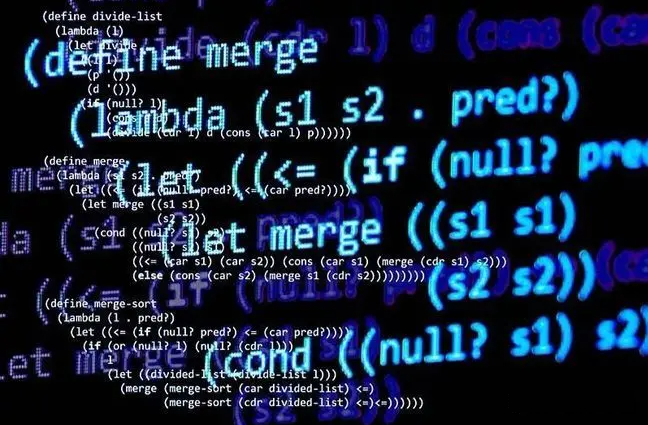Once you have learned how to write functions without side effects, the new problem we have to solve is how to combine these functions.

For example, the `sqr2 function` above has a problem. If the input is not of number type, the computing will go wrong. According to the imperative idea, we may directly change the code of sqr2 to the code below:

``````let sqr2 = function(x){
if (typeof x === 'number'){
return x * x;
}else{
return 0;
}
}``````

However, the code of sqr2 has been tested. Can we judge outside instead of changing it?

Yes, we can write it like this:

``````let isNum = function(x){
if (typeof x === 'number'){
return x;
}else{
return 0;
}
}
console.log(sqr2(isNum("20")));``````

Alternatively, we can reserve a position for the preprocessing function when designing sqr2 and change it if we want to upgrade in the future, with the main logic unchanged:

``````let sqr2_v3 = function(fn, x){
let y = fn(x);
return y * y;
}
console.log((sqr2_v3(isNum,1.1)));``````

If you think it annoying to write isNum every time, you can define a new function and make isNum fixed:

``````let sqr2_v4 = function(x){
return sqr2_v3(isNum,x);
}
console.log(Math.sin(undefined));``````

## 5. Encapsulate Functional Capablities with Containers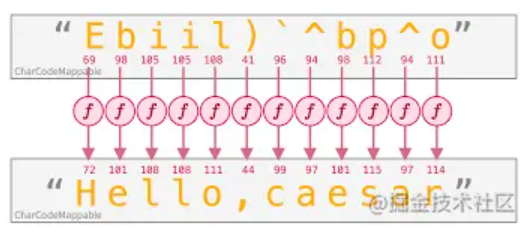Now, if we want to reuse the isNum capability for sqr2 and other mathematical functions.

For example, if you compute undefined for Math.sin, you will get a NaN:

``console.log(Math.sin(undefined));``

At this time, we need to use an object-oriented idea to encapsulate isNum's capabilities into a class:

``````class MayBeNumber{
constructor(x){
this.x = x;
}

map(fn){
return new MayBeNumber(fn(isNum(this.x)));
}

getValue(){
return this.x;
}
}``````

As such, no matter which object we get, we can use it to construct a MayBeNumber object and then call the map method of this object to call mathematical functions. Thus, we have the isNum capability.

Let's look at one example of calling sqr2:

``````let num1 = new MayBeNumber(3.3).map(sqr2).getValue();
console.log(num1);
let notnum1 = new MayBeNumber(undefined).map(sqr2).getValue();
console.log(notnum1);``````

We can replace sqr2 with Math.sin:

``````let notnum2 = new MayBeNumber(undefined).map(Math.sin).getValue();
console.log(notnum2);``````

The output has changed from NaN to 0.

A benefit of encapsulating into objects is that we can use "." to call it many times. For example, if we want to call it twice to get a quartic, we only need another `. map(sqr2)` after `. map(sqr2)`.

``````let num3 = new MayBeNumber(3.5).map(sqr2).map(sqr2).getValue();
console.log(num3);``````

Another benefit of encapsulating into objects is that functional nested calls are in reverse order of the imperative ones, while the map is in the same order.

If you cannot understand, here is an example. If we want to calculate the square of sin(1) using functional calls, we must write sqr2 (which will be executed next) and then write Math.sin (which will be executed first):

``console.log(sqr2(Math.sin(1)));``

Calling map is the same as imperative:

``````let num4 = new MayBeNumber(1).map(Math.sin).map(sqr2).getValue();
console.log(num4);``````

## 6. Encapsulate "New" With "Of"Encapsulating into an object looks good, but functional programming also creates new objects and maps. Why not use a function when constructing an object?

This is easy, so let's define an "of" method for it:

``````MayBeNumber.of = function(x){
return new MayBeNumber(x);
}``````

Now, we can use an "of" method to construct the MayBeNumber object:

``````let num5 = MayBeNumber.of(1).map(Math.cos).getValue();
console.log(num5);
let num6 = MayBeNumber.of(2).map(Math.tan).map(Math.exp).getValue();
console.log(num6);``````

We can also upgrade the map function using an "of" method.

The previous isNum has a problem. If the input is not a number, it is not necessary to assign 0 to call the function. We can return 0 directly.

We haven't written any arrow function before, so we can give it a try here:

``isNum2 = x => typeof x === 'number';``

Rewrite the map with isNum2 and the “of” method:

``````    map(fn){
if (isNum2(this.x)){
return MayBeNumber.of(fn(this.x));
}else{
return MayBeNumber.of(0);
}
}``````

Let's look at another situation. When we process the return value, if there is an error, we will not process the return value that is Ok. We can write this way:

``````lass Result{
constructor(Ok, Err){
this.Ok = Ok;
this.Err = Err;
}

isOk(){
return this.Err === null || this.Err === undefined;
}

map(fn){
return this.isOk() ? Result.of(fn(this.Ok),this.Err) : Result.of(this.Ok, fn(this.Err));
}
}
Result.of = function(Ok, Err){
return new Result(Ok, Err);
}

console.log(Result.of(1.2,undefined).map(sqr2));``````

The output is listed below:

``Result { Ok: 1.44, Err: undefined }``

Let's summarize the design pattern of the previous container:

1. There is a container for storing values.
2. This container provides a map function, whose function is to compute the value in the container with the function that map function calls. The object returned eventually is still an object of the container.

We can call this design pattern Functor.

If the container also provides an “of” function to convert values into containers, it is called Pointed Functor.

For example, let's look at the Array type in JavaScript:

``````let aa1 = Array.of(1);
console.log(aa1);
console.log(aa1.map(Math.sin));``````

It supports the “of” function and the map function to call Math.sin to compute the value in Array. The result of map function's calculation is still an Array.

Then, we can say that Array is a Pointed Functor.

## 7. Simplify the Object Level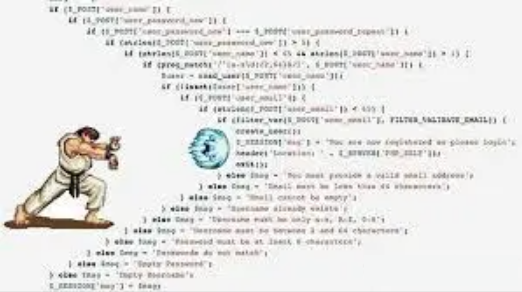Our functions are upgraded accordingly with the Result structure above. If it is a numeric value, Ok is a numeric value and Err is undefined. If it is not a numeric value, Ok is undefined and Err is 0:

``````let sqr2_Result = function(x){
if (isNum2(x)){
return Result.of(x*x, undefined);
}else{
return Result.of(undefined,0);
}
}``````

We can call this new sqr2_Result function:

``console.log(Result.of(4.3,undefined).map(sqr2_Result));``

A nested result is returned:

``Result { Ok: Result { Ok: 18.49, Err: undefined }, Err: undefined }``

We need to add a join function to the Result object to get the value of the sub-Result to the parent Result:

``````    join(){
if (this.isOk()) {
return this.Ok;
}else{
return this.Err;
}
}``````

When we call, add the join function at the end:

``console.log(Result.of(4.5,undefined).map(sqr2_Result).join());``

The result of nesting becomes one level:

``Result { Ok: 20.25, Err: undefined }``

It takes time to write map(fn).join() each time we call . We can define a flatMap function to handle it once and for all:

``````    flatMap(fn){
return this.map(fn).join();
}``````

We call it this way:

``console.log(Result.of(4.7,undefined).flatMap(sqr2_Result));``

Result:

``Result { Ok: 22.090000000000003, Err: undefined }``

Let's finally take an overall look at this Result class:

``````class Result{
constructor(Ok, Err){
this.Ok = Ok;
this.Err = Err;
}

isOk(){
return this.Err === null || this.Err === undefined;
}

map(fn){
return this.isOk() ? Result.of(fn(this.Ok),this.Err) : Result.of(this.Ok, fn(this.Err));
}

join(){
if (this.isOk()) {
return this.Ok;
}else{
return this.Err;
}
}

flatMap(fn){
return this.map(fn).join();
}
}
Result.of = function(Ok, Err){
return new Result(Ok, Err);
}``````

A Pointed Functor like Result, which implements the flatMap functionality, is the so-called Monad.

## 8. Partial Functions and Higher-Order Functions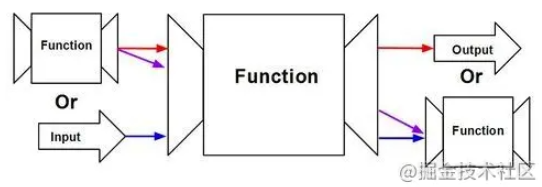We are quite familiar with the usage of functions in the previous functional programming modes. Let's summarize the biggest difference in experience between functional programming and command line programming:

1. Functions are first-class formulas. We should be familiar with saving functions in variables before calling them.
2. Functions can appear in return values. The most important usage is to convert functions with n(n>2) parameters input into n series calls with 1 parameter. This is called currying. This new function with reduced parameters is what we call the partial function.
3. Some functions can be used as the parameters of functions. They are called higher-order functions.

The partial function can be regarded as a more flexible default value of parameters.

For example, there is a structure called spm, which consists of spm_a and spm_b. However, spm_a is fixed in a module. Most of the time, only spm_b needs to be specified. So, we can write a partial function:

``````const getSpm = function(spm_a, spm_b){
return [spm_a, spm_b];
}

const getSpmb = function(spm_b){
return getSpm(1000, spm_b);
}

console.log(getSpmb(1007));``````

Higher-order functions are already well-used in the previous map and flatMap. However, there are still many design patterns worth learning about higher-order functions.

Consider how to implement an effective function that only executes once in a functional way.At the same time, don't use context variables, which is not a functional idea. We need closures here.

once is a higher-order function, and the return value is a function. If done's value is false, set it to true and then execute fn. Done is in the same layer of the returned function, so it will be obtained by closure memory:

``````const once = (fn) => {
let done = false;
return function() {
return done ? undefined : ((done=true), fn.apply(this,arguments));
}
}

let init_data = once(
() => {
console.log("Initialize data");
}
);

init_data();
init_data();``````

We can see that nothing happened to the second call of init_data().

## 9. Recursion and Memory

A lot has been introduced already, but functional programming is quite complicated since it involves recursion.

The simplest thing in recursion is factorial:

``````let factorial = (n) => {
if (n===0){
return 1;
}
return n*factorial(n-1);
}

console.log(factorial(10));``````

We all know that this is very inefficient and will bring a lot of repetitive computing. We need to adopt the method of dynamic programming.

How can we use dynamic planning in functional programming? How can we save the values that have been computed?

After reading the previous section, the first thing that comes to your mind may be using closures. Yes, we can encapsulate a higher-order function called memo to achieve this function:

``````const memo = (fn) => {
const cache = {};
return (arg) => cache[arg] || (cache[arg] = fn(arg));
}``````

The logic here is very simple. The return value is a Lambda expression, which still supports closures. So, we define a cache at its same layer and then compute and save an item in the cache if it is empty. We can also use it directly if the item already exists.

This high-order function is very easy to use and the factorial logic does not need to be changed. Put it in the memo:

``````let fastFact = memo(
(n) => {
if (n<=0){
return 1;
}else{
return n * fastFact(n-1);
}
}
);``````

``const memoizedValue = useMemo(() => computeExpensiveValue(a, b), [a, b]);``

## 10. Summary

1. The core concept of functional programming is very simple. It stores functions in variables and uses them in parameters and return values.
2. When programming, always remember to separate code with side effects from code without side effects.
3. The principle of functional programming is very simple. However, since we are used to imperative programming, there will be many difficulties at the beginning. You will feel more comfortable with functional programming after practicing a lot.
4. Functional programming has a mathematical foundation. For now, we can learn it as a design pattern. However, we suggest knowing the real principle behind it after getting familiar with it in the future.
0 0 0
Share on

# Related Products

• ##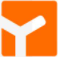YiDA Low-code Development Platform

A low-code development platform to make work easier

• ##mPaaS

Help enterprises build high-quality, stable mobile apps

• ##Web Hosting

Explore how our Web Hosting solutions help small and medium sized companies power their websites and online businesses.